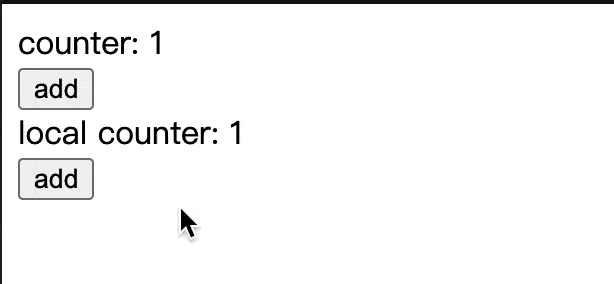# 使用 Model#

## 在组件内使用#

### 作为全局状态使用#

`useModel` 支持传入多个 Model，多个 Model 的 State 和 Actions 会进行合并后作为返回结果。例如：

``````const fooModel = model('foo').define({
state: {
value: 1,
},
actions: {
state += 1;
},
},
});

const barModel = model('bar').define({
state: {
title: 'bar',
},
actions: {
},
},
});

const [state, actions] = useModel([fooModel, barModel]);
// 或
const [state, actions] = useModel(fooModel, barModel);``````

`state``actions` 的值分别为：

``````state = {
value: 1,
title: 'bar',
};

actions = {
state += 1;
},
},
};``````

`useModel` 还支持对 State 和 Actions 做 selector 操作，实现对 State 和 Actions 的筛选或重命名，例如：

``````const fooModel = model('foo').define({
state: {
value: 1,
},
actions: {
state += 1;
},
},
});

const barModel = model('bar').define({
state: {
value: 'bar',
},
actions: {
},
},
});

const [state, actions] = useModel(
[fooModel, barModel],
(fooState, barState) => ({
fooValue: fooState.value,
barValue: barState.value,
}), // stateSelector
);``````

``````const [state, actions] = useModel(
[fooModel, barModel],
undefined,
);``````

### 作为静态状态使用#

INFO

`useStaticModel` 的使用方式和 `useModel` 完全一致。

``````import { useStaticModel } from '@modern-js/runtime/model';

function Search() {
// 这里注意不要解构 state
const [state] = useStaticModel(searchModel);

return (
<div>
<button
onClick={async () => {
const result = await mockSearch(state.input);
console.log(result);
}}
>
Search
</button>
</div>
);
}``````

`useStaticModel` 还适合和 react-three-fiber 等动画库一起使用，因为在动画组件 UI 里绑定会快速变化的状态，容易引起性能问题。这种情况就可以选择使用 `useStaticModel`，它只会订阅状态，但不会引起视图组件的重新渲染。下面是一个简化示例：

``````function ThreeComponent() {
const [state, actions] = useStaticModel(modelA);

useFrame(() => {
state.value; // 假设初始化为 0
actions.setValue(1);
state.value; // 这里会得到1
});
}``````

### 作为局部状态使用#

INFO

`useLocalModel` 的使用方式和 `useModel` 完全一致。

``````import { useLocalModel } from '@modern-js/runtime/model';

function LocalCounter() {
const [state, actions] = useLocalModel(countModel);

return (
<div>
<div>local counter: {state.value}</div>
</div>
);
}``````## 在组件外使用#

``````let store; // 组件外部 `store` 对象的引用
function setStore(s) {
store = s;
}
function getStore() {
return store;
}

function Counter() {
const [state] = useModel(countModel);
const store = useStore();
// 通过 useMemo 避免不必要的重复设置
useMemo(() => {
setStore(store);
}, [store]);

return (
<div>
<div>counter: {state.value}</div>
</div>
);
}``````

``````setInterval(() => {
const store = getStore();
const [, actions] = store.use(countModel);